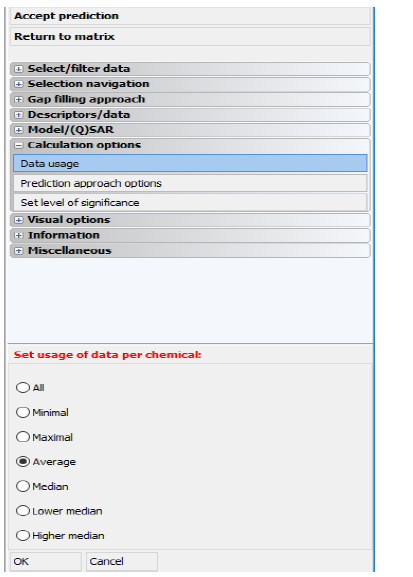The following examples describe different approaches for usage of data and making predictions in categorical scales. They are prepared for imaginary scale containing four possible values and three chemicals having more than one observed value for the selected endpoint.

The first picture represents the real observed values for the three chemicals.

1. DataUsage All, all points will be taken into the calculations.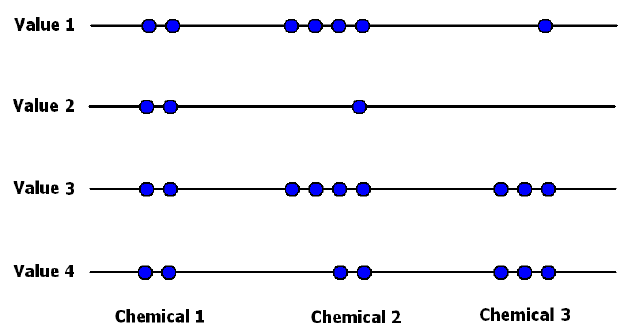Fig. 1 The real observed values for chemicals (the same picture for using all data points)

The next pictures represent the recalculated values for the three chemicals. The real observed values are blank and the recalculated values are blue.

2. DataUsage Minimal, one point per chemical is given.Fig. 2 The recalculated values for chemicals (when using minimal value for each chemical)

3) DataUsage Maximal, one point per chemical is given.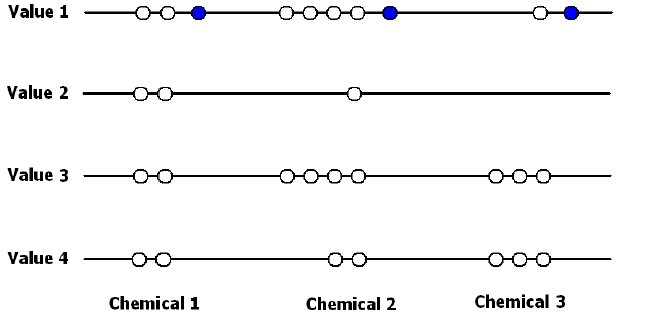Fig. 3 The recalculated values for chemicals (when using maximal value for each chemical)

4) DataUsage Median(s), Chemicals 1 and 2 have two medians; Chemical 3 has one median (value 2 is not taken into account here).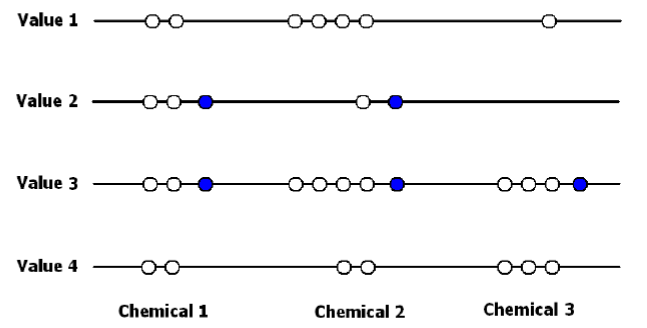Fig. 4 The recalculated values for chemicals (when using median values for each chemical)

5) DataUsage Lower median, one point per chemical is given.Fig. 5 The recalculated values for chemicals (when using lower median value for each chemical)

6) DataUsage Higher median, one point per chemical is given.Fig. 6 The recalculated values for chemicals (when using higher median value for each chemical)

7) DataUsage=Mode(s), Chemicals 2 and 3 have two modes; Chemical 1 has four modes.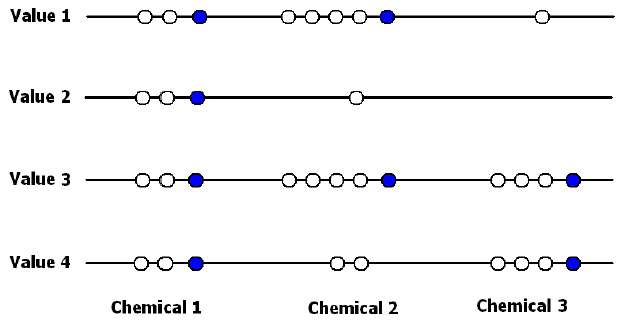Fig. 7 The recalculated values for chemicals (when using mode values for each chemical)

8) DataUsage Lowest mode, one point per chemical is given.Fig. 8 The recalculated values for chemicals (when using lowest mode value for each chemical)

9) DataUsage Highest mode, one point per chemical is given.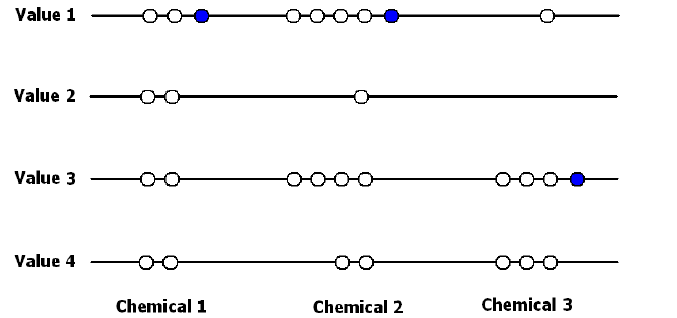Fig. 9 The recalculated values for chemicals (when using highest mode value for each chemical)

Making predictions

Let’s assume that Chemical 2 and 3 are the neighbors that determine the prediction. The various cases shown above will look as follows:

1)  DataUsage All, the prediction value is:

- Value 4, when the approximation type is “Minimal”

- Value 1, when the approximation type is “Maximal”

- No value, when the approximation type is “Median” – Value2 and Value 3 are both medians, so the system cannot make a decision automatically

- Value 3, when the approximation type is “Lower median”

- Value 2, when the approximation type is “Higher median”

- Value 3, when the approximation type is “Mode”, “Lowest mode” or “Highest mode” – 7 neighbor points are available for this value; only one mode value is available in this case, so the last three approximation types give the same prediction value.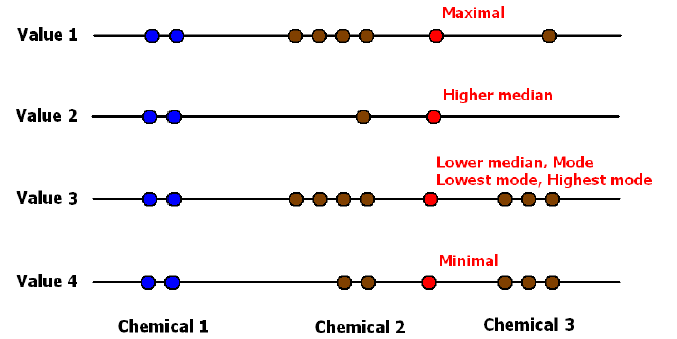Fig. 10 The prediction values when using all data points

2)  DataUsage=Minimal, the prediction value is:

- Value 4, for all approximation types.Fig. 11 The prediction value when using minimal value for each chemical

3)  DataUsage Maximal, the prediction value is:

- Value 1, for all approximation types.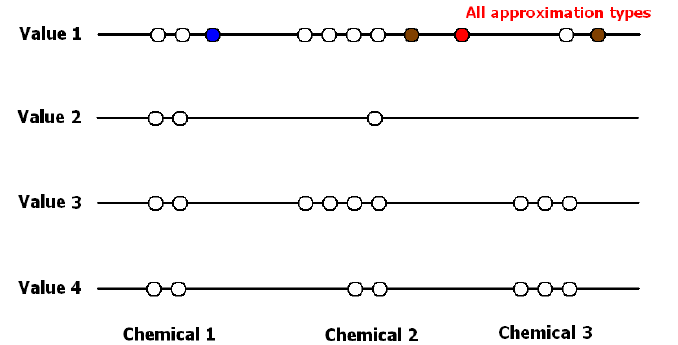Fig. 12 The prediction value when using maximal value for each chemical

4)  DataUsage=Median(s), the prediction value is:

- Value 3, when the approximation type is “Minimal”

- Value 2, when the approximation type is “Maximal”

- No value, when the approximation type is “Median” – Value2 and Value 3 are both medians, so the system cannot make a decision automatically

- Value 3, when the approximation type is “Lower median”

- Value 2, when the approximation type is “Higher median”

-Value 3, when the approximation type is “Mode”, “Lowest mode” or “Highest mode” – 2 neighbor points are available for this value; only one mode value is available in this case, so the last three approximation types give the same prediction value.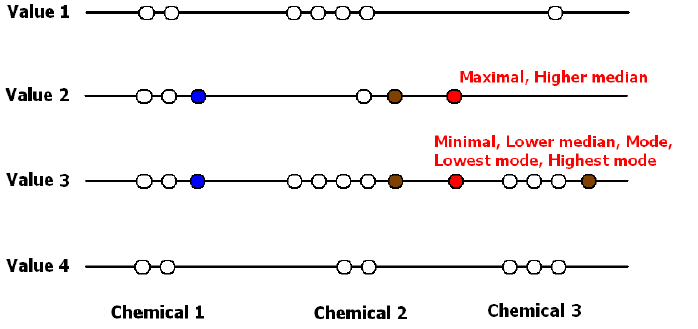Fig. 13 The prediction values when using median values for each chemical

5)  DataUsage=Lower median, the prediction value is:

- Value 3, for all approximation types.Fig. 14 The prediction value when using lower median value for each chemical

6)  DataUsage=Higher median, the prediction value is:

- Value 3, when the approximation type is “Minimal”

- Value 2, when the approximation type is “Maximal”

- No value, when the approximation type is “Median” – Value2 and Value 3 are both medians, so the system cannot make a decision automatically

- Value 3, when the approximation type is “Lower median”

- Value 2, when the approximation type is “Higher median”

- No value, when the approximation type is “Mode” – Value2 and Value 3 are both modes, so the system cannot make a decision automatically

- Value 3, when the approximation type is “Lowest mode”

- Value 2, when the approximation type is “Highest mode”Fig. 15 The prediction value when using higher median value for each chemical

7)  DataUsage=Mode(s), the prediction value is:

- Value 4, when the approximation type is “Minimal”

- Value 1, when the approximation type is “Maximal”

- Value 3, when the approximation type is “Median”, “Lower median” and “Higher median” – only one median value is available in this case, so these three approximation types give the same prediction value

-Value 3, when the approximation type is “Mode”, “Lowest mode” or “Highest mode” – 2 neighbor points are available for this value; only one mode value is available in this case, so the last three approximation types give the

same prediction value.Fig. 16 The prediction values when using mode values for each chemical

8)  DataUsage=Lowest mode, the prediction value is:

- Value 4, when the approximation type is “Minimal”

- Value 3, when the approximation type is “Maximal”

- No value, when the approximation type is “Median” – Value 3 and Value 4 are both medians, so the system cannot make a decision automatically

- Value 4, when the approximation type is “Lower median”

- Value 3, when the approximation type is “Higher median”

- No value, when the approximation type is “Mode” – Value 3 and Value 4 are both modes, so the system cannot make a decision automatically

- Value 4, when the approximation type is “Lowest mode”

- Value 3, when the approximation type is “Highest mode”.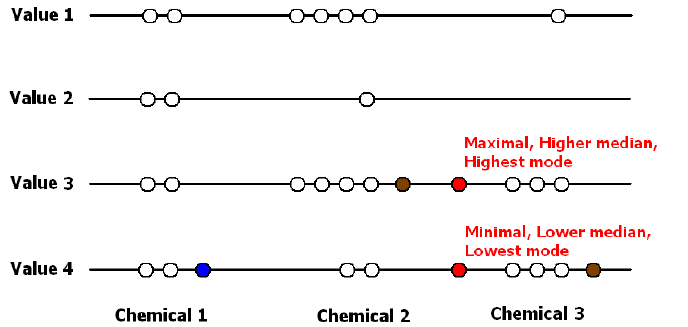Fig. 17 The prediction value when using lowest mode value for each chemical

9)  DataUsage=Highest mode, the prediction value is:

- Value 3, when the approximation type is “Minimal”

- Value 1, when the approximation type is “Maximal”

- No value, when the approximation type is “Median” – Value 1 and Value3 are both medians (Value 2 is not taken into account here), so the system cannot make a decision automatically

- Value 3, when the approximation type is “Lower median”

- Value 1, when the approximation type is “Higher median”

- No value, when the approximation type is “Mode” – Value 1 and Value 3 are both modes, so the system cannot make a decision automatically

- Value 3, when the approximation type is “Lowest mode”

- Value 1, when the approximation type is “Highest mode”.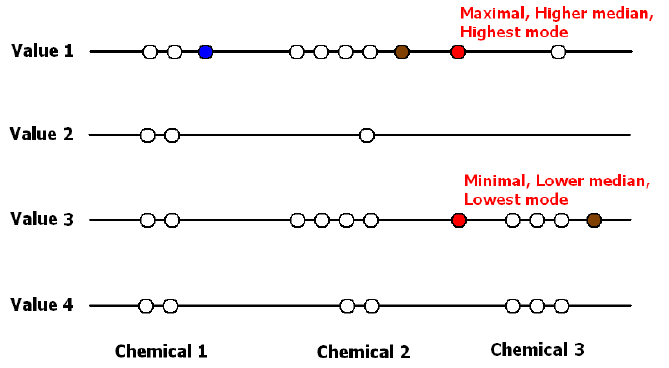Fig. 18 The prediction value when using highest mode value for each chemical